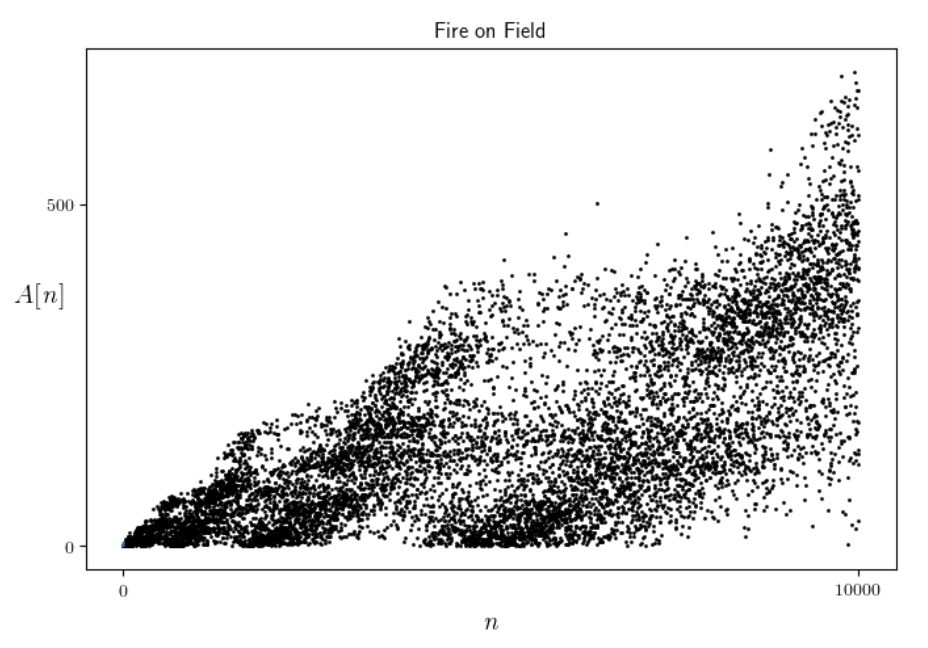시간 제한메모리 제한제출정답맞힌 사람정답 비율
1 초 512 MB55736932767.702%

## 문제

We define A as a sequence of positive integers like the following.

Let A = 1, A = 1. For a positive integer i ≥ 2, A[i] is the smallest positive integer under the condition that no three terms A[i − 2k], A[ik], and A[i] form an arithmetic progression for any integer k > 0 such that i − 2k ≥ 0, that is, A[i] − A[ik] ≠ A[ik] − A[i − 2k].

The sequence is uniquely determined like the following sequence: A = 1, A = 1, A = 2, A = 1, A = 1, A = 2, A = 2, A = 4, A = 4, etc. The sequence element A cannot be 1 since otherwise A = 1, A = 1, A = 1 form an arithmetic progression; here i = 2 and k = 1. If A is any integer larger than one, then the condition is satisfied. Therefore, A should be 2 which is the smallest positive integer among the possible ones. Similarly, it is easy to know that A = 1. The sequence element A cannot be 3 since otherwise A − A[4 − 2] = A[4 − 2] − A[4 − 2 × 2] ; here i = 4 and k = 2 . Other natural numbers like 1, 2 and 4 are also possible for A, but the smallest one is 1. Therefore, A = 1.

This sequence is called “fire on field” or “forest fire” since the scatter plot of the sequence looks like spreading fire on a field. See the figure below.Given a non-negative integer n, write a program to output A[n].

## 입력

Your program is to read from standard input. The input consists of one line containing one non-negative integer n (0 ≤ n ≤ 1,000).

## 출력

Your program is to write to standard output. Print exactly one line. The line should contain A[n].

## 예제 입력 1

5


## 예제 출력 1

2


## 예제 입력 2

8


## 예제 출력 2

4


## 예제 입력 3

100


## 예제 출력 3

4